• 可以用于LSTM预测数据，和权重更新程序已上传
• ## 深度学习-LSTM预测未来值

千次阅读 多人点赞 2021-01-22 14:48:04
LSTM进行输出预测 ** 本文基于LSTM使用六小时（360分钟）的数据预测10分钟后的发电量，主要就是讲解一下写代码过程中遇到的一些不太懂的地方，供同为萌新的参考，已经很了解深度学习的话，可以直接关闭了23333...
**
用LSTM进行多输出预测
** 本文基于LSTM使用六小时（360分钟）的数据来预测10分钟后的发电量，主要就是讲解一下写代码过程中遇到的一些不太懂的地方，供同为萌新的参考，已经很了解深度学习的话，可以直接关闭了23333。本篇中使用的数据是经过数据筛选和处理的，有关数据筛选和处理的部分后续会上传。
1.参数列表
为了方便在不同情境下进行调参，易于其他人的使用，习惯性把一些需要修改的参数前置。首先选择读入文件的路径和文件名，再修改程序的工作路径到你文件所存储的位置（os.chdir(SaveFile_Path)），此处之所以要修改工作路径的原因是如果不加这条会默认程序执行在你程序所处的位置，后续存储文件时如果使用相对路径，会直接存储到python程序所在文件夹，如果对这个不介意这段可不加。*记得在输入存储位置时加上转义符r’’，否者会判定\为保留字。*然后再创建一个文件夹存储你所得到的那些结果，用if判断一下文件夹是否存在，如果不存在则创建一个。
'''修改数据'''
SaveFile_Path = r'D:\Desktop\2018年5MW运行数据\2018年\随机森林挑选特征'  # 要读取和保存的文件路径
savename = r'201803-12特征量.csv'
os.chdir(SaveFile_Path)  # 修改当前工作目录

DIR = 'LSTM'
if not os.path.exists(DIR):  #判断括号里的文件是否存在的意思，括号内的可以是文件路径。
os.makedirs(DIR)  #用于递归创建目录

TIME_STEP = 360 #时间步长360min 6h
DELAY = 10 #预测十分钟后
BATCHSZ = 5  #一个批处理(batch)大小
EACH_EPOCH = 3  #循环次数

2.绘制初始曲线
这部分没什么好说的，就是将你的数据绘制成图像，观察大致趋势。
'''
绘制初始数据曲线
'''
def plot_init():
values = df.values
col = df.shape
plt.rcParams['font.sans-serif'] = ['SimHei']  # 用来正常显示中文标签
plt.figure()
for i in range(0, col):
plt.subplot(col, 1, i+1)
plt.plot(values[:, i])
plt.title(df.columns[i], y=0.5, loc='right')
plt.show()

3.LSTM数据准备
送入LSTM网络的数据格式必须是（样本数，时间步长，特征量），所以我们需要将数据处理一下。这里需要注意的是需要进行归一化，否则收敛困难。本文使用360min的数据去预测10min后的数据，总共21万行数据，20万用作训练，1万行作测试集，没有设置验证集。从数据中划分出标签，应为时间步长360min后的连续10min，接着时间步长窗口和标签窗口逐行滑动，生成LSTM的输入数据和标签。训练集使用随机种子进行伪随机打乱。函数返回train_X, train_y, test_X, test_y, scaler。
我在写的时候有疑惑的几个点： fit_transform()的作用,与fit()、transform()的区别，这里简单介绍一下fit_transform()的作用，是对这部分数据先拟合，找到该part的整体指标，如均值、方差、最大值、最小值等等，然后对该trandata进行转换transform，从而实现数据的标准化、归一化，它是fit()、transform()的组合。之后可以用scaler.inverse_transform()将归一化的数据还原回去。
tf.random.set_seed(7)最开始我将import tensorflow as tf放在import部分的最下面报错说tensorflow中无random模块，之后将import tensorflow as tf往前移了几个错误消失，具体原因现在还没搞清楚，我觉得是前面导入模块对import tensorflow的影响。
'''
LSTM数据准备
'''

def train_test(timestep, nextstep):   #timestep时间步长, nextstep预测多长时间
values = dataset.values
# ensure all data is float
values = values.astype('float32')
# normalize features归一化
scaler = MinMaxScaler(feature_range=(0, 1))  # sklearn 归一化函数
scaled = scaler.fit_transform(values)  # fit_transform(X_train) 意思是找出X_train的均值和​​​​​​​标准差，并应用在X_train上。
# split into train and test sets  将数据集进行划分，然后将训练集和测试集划分为输入和输出变量，最终将输入（X）改造为LSTM的输入格式，即[samples,timesteps,features]
n_train_hours = 200000 #20万行作训练集，1万作测试集
train = scaled[:n_train_hours, :]
test = scaled[n_train_hours:, :]

train_X = []
train_y = []
test_X = []
test_y = []

# 测试集：
# 利用for循环，遍历整个测试集，提取测试集中连续360min的特征量作为输入特征test_X，第361-370min的发电量作为标签
for i in range(len(train)-timestep-nextstep+1):
train_X.append(train[i:(i+timestep), :])
btemp = train[i+timestep:i+timestep+nextstep, 0]
b = []
for j in range(len(btemp)):
b.append(btemp[j])
train_y.append(b)

# 对训练集进行打乱
np.random.seed(7)
np.random.shuffle(train_X)
np.random.seed(7)
np.random.shuffle(train_y)
tf.random.set_seed(7)
# 将训练集由list格式变为array格式
train_X = np.array(train_X, dtype=np.float32)
train_y = np.array(train_y, dtype=np.float32)

# 使x_train符合RNN输入要求：[送入样本数， 循环核时间展开步数， 每个时间步输入特征个数]。
# 此处整个数据集送入，送入样本数为train_X.shape；输入360min数据，预测出第361min的发电量，循环核时间展开步数为360; 每个时间步送入的特征是某一min的运行数据，16个数据，故每个时间步输入特征个数为16
train_X = np.reshape(train_X, (train_X.shape, 360, 16))

# 测试集：
# 利用for循环，遍历整个测试集，提取测试集中连续360min的特征量作为输入特征test_X，第361-370min的发电量作为标签
for i in range(len(test)-timestep-nextstep+1):
test_X.append(test[i:(i + timestep), :])
btemp = test[i + timestep:i + timestep + nextstep, 0]
b = []
for j in range(len(btemp)):
b.append(btemp[j])
test_y.append(b)

test_X, test_y = np.array(test_X, dtype=np.float32), np.array(test_y, dtype=np.float32)
test_X = np.reshape(test_X, (test_X.shape, 360, 16))

#print(train_X.shape, train_y.shape, test_X.shape, test_y.shape)
return train_X, train_y, test_X, test_y, scaler

4.构造模型
模型构造这一块比较简单，主要是四个LSTM层再加一层全连接层，中间加了几个Dropout防止过拟合，神经元个数可以根据自己的需求进行调整，神经元个数越多，计算量越大。
def model_build(train_datas): #train_datas = train_X
# LSTM层
model = keras.models.Sequential()
model.add(keras.layers.LSTM(40, input_shape=(train_datas.shape[1:]), return_sequences=True, )) #, return_sequences=True 400记忆体个数
#配置训练方法

return model

5.模型拟合
这里主要讲一下添加的一些函数， model.load_weights(checkpoint_save_path) 先判断是否有训练过的模型，如果有加载模型继续进行训练。 keras.callbacks.ReduceLROnPlateau patience=4，连续4个batch_size损失函数没有变化就会调整学习率，新的学习率=旧的学习率*factor，min_lr当学习率小于这个数值时，循环停止。 keras.callbacks.ModelCheckpoint存储模型。 keras.callbacks.EarlyStopping连续15个batch_size损失函数没有变化，循环停止。
'''模型拟合'''
def model_fit(model, train_datas, train_labels,x_test, y_test):    #train_X, train_y, test_X, test_y

checkpoint_save_path = "./checkpoint/LSTM_stock.ckpt" #模型保存位置

if os.path.exists(checkpoint_save_path + '.index'):

lr_reduce = keras.callbacks.ReduceLROnPlateau('val_loss',     #学习停止，模型会将学习率降低2-10倍，该hui
patience=4,
factor=0.7,
min_lr=0.00001)
best_model = keras.callbacks.ModelCheckpoint(filepath=checkpoint_save_path,#保存模型
monitor='val_loss',
verbose=0,
save_best_only=True,
save_weights_only=True,
mode='min',
)

early_stop = keras.callbacks.EarlyStopping(monitor='val_rmse', patience=15)

history = model.fit(
train_datas, train_labels,
validation_data=(x_test, y_test),
batch_size=BATCHSZ,
epochs=EACH_EPOCH,
verbose=2,
callbacks=[
best_model,
early_stop,
lr_reduce,
]
)

loss = history.history['loss']
val_loss = history.history['val_loss']
#画出训练集和测试集的损失函数
plt.plot(loss, label='Training Loss')
plt.plot(val_loss, label='Validation Loss')
plt.title('Training and Validation Loss')
plt.legend()
plt.savefig('./{}/{}.png'.format(DIR, '损失函数'))
plt.close()

return model, history

问题：编写代码时无法使用keras模型中的model.save(‘my_model.hdf5’)一直报错a bytes-like object is required, not ‘str’。这个问题我现在还没搞清是因为什么，所以我这里选择不使用它。
6.评价部分
评价部分主要使用两个指标，RMSE和R2_score。数据在之前已经进行过归一化，所以如果想还原真实的大小，需要使用scaler.inverse_transform()。关于这个函数有个小tip就是，需要把数据还原成原来使用fit_transform()时的shape，比如这里是二维的数据使用fit_transform()，那我使用scaler.inverse_transform()的数据列数shape必须与前面的数据一致，shape可以不一致。举个简单的例子：
import numpy as np
from sklearn.preprocessing import MinMaxScaler

data = np.array([
[1, 8],
[2, 7],
[3, 6],
[4, 7],
])
scaler = MinMaxScaler(feature_range=(0, 1))  # sklearn 归一化函数
scaled = scaler.fit_transform(data)
d2 = np.array([
[0.2, 0.3],
[0.1, 0.4]
])
d3 = scaler.inverse_transform(d2)
print(scaled)
print(d3)


d2和data的shape一致，可以使用scaler.inverse_transform（）
[[0.         1.        ]
[0.33333333 0.5       ]
[0.66666667 0.        ]
[1.         0.5       ]]
[[1.6 6.6]
[1.3 6.8]]

这里我为了方便画图和进行数据分析，只取了预测的十个数据中的最后一个，也就是说我只关注预测的第10min的数据。
'''评价部分'''
def rmse(y_true, y_pred):  #sqrt求元素平方根  mean求张量平均值
return keras.backend.sqrt(keras.backend.mean(keras.backend.square(y_pred - y_true), axis=-1))

def model_evaluation(model, test_X, test_y, savename):
yhat = model.predict(test_X)
yhat = yhat[:, -1]    #只取最后一个时刻的进行分析，1-9min的结果不是很重要
y_normal = yhat.copy()
y_normal[y_normal < 0] = 0  #将预测值归一化中小于0的都置0
test_X = test_X[:, 0, :]
test_y = test_y[:, -1]   #对应取test_y最后一个时刻

#画出归一化后真实值和预测值的图像计算相关指标
model_plot(test_y, y_normal, '归一化')
RMSE_normal = np.sqrt(mean_squared_error(y_normal, test_y))
R2_SCORE_normal = r2_score(y_normal, test_y)
print('RMSE_normal: {}\nR2_SCORE_normal: {}\n'.format(RMSE_normal, R2_SCORE_normal))

#返回计算归一化前
yhat = yhat.reshape(len(yhat), 1)
inv_yhat = concatenate((yhat, test_X[:, 1:]), axis=1)
inv_y = scaler.inverse_transform(inv_yhat)  # 预测值转化
y_pred = inv_y[:, 0]   #预测值由归一化值转为真实值
y_pred[y_pred < 0] = 0  #将预测值真实值中小于0的都置0

test_y = test_y.reshape((len(test_y), 1))
inv_yact_hat = concatenate((test_y, test_X[:, 1:]), axis=1)
inv_y = scaler.inverse_transform(inv_yact_hat)
y_real = inv_y[:, 0]  # 标签由归一化值转为真实值
'''
在这里为什么进行比例反转，是因为我们将原始数据进行了预处理（连同输出值y），
此时的误差损失计算是在处理之后的数据上进行的，为了计算在原始比例上的误差需要将数据进行转化。
'''

#存储真实值和预测值的相关信息
y_pred_df = pd.DataFrame(index=y_pred)
y_pred_df.to_csv(r'./{}/LSTM_pred.csv'.format(DIR), encoding='gbk', sep=',')
y_real_df = pd.DataFrame(index=y_real)
y_real_df.to_csv(r'./{}/LSTM_real.csv'.format(DIR), encoding='gbk', sep=',')

# 画出真实值和预测值的图像并计算相关指标
model_plot(y_real, y_pred, savename)
RMSE = np.sqrt(mean_squared_error(y_pred, y_real))
R2_SCORE = r2_score(y_pred, y_real)
print('RMSE: {}\nR2_SCORE: {}\n'.format(RMSE, R2_SCORE))

return RMSE, R2_SCORE, RMSE_normal, R2_SCORE_normal

7.保存模型信息和绘图
这部分没什么难点，比较简单，就是存储一下运行的结果。
'''保存模型信息'''
def model_save(RMSE, R2_SCORE, RMSE_normal, R2_SCORE_normal, savename):
with open(r'./{}/LSTM.txt'.format(DIR), 'a') as fh:
fh.write('参数设置：\nTIME_STEP: {}\tDELAY: {}\n'.format(TIME_STEP, DELAY))
fh.write('RMSE: {}\nR2_SCORE: {}\n'.format(RMSE, R2_SCORE))
fh.write('RMSE_normal: {}\nR2_SCORE: {}\n\n'.format(RMSE_normal, R2_SCORE_normal))
print('%s模型信息保存成功！\n\n\n' % savename)

'''绘图相关'''
def model_plot(y_real, y_pred, savename):
plt.cla()
fig1 = plt.figure(figsize=(10, 14), dpi=80)

ax1 = fig1.add_subplot(1, 1, 1)  # 1行x1列的网格里第一个
ax1.plot(y_real, '-', c='blue', label='Real', linewidth=2)
ax1.plot(y_pred, '-', c='red', label='Predict ', linewidth=2)
ax1.legend(loc='upper right')
ax1.set_xlabel('min')
ax1.set_ylabel('KWH')
ax1.grid()

fig1.savefig('./{}/{}.png'.format(DIR, savename))
plt.close()

8.结果部分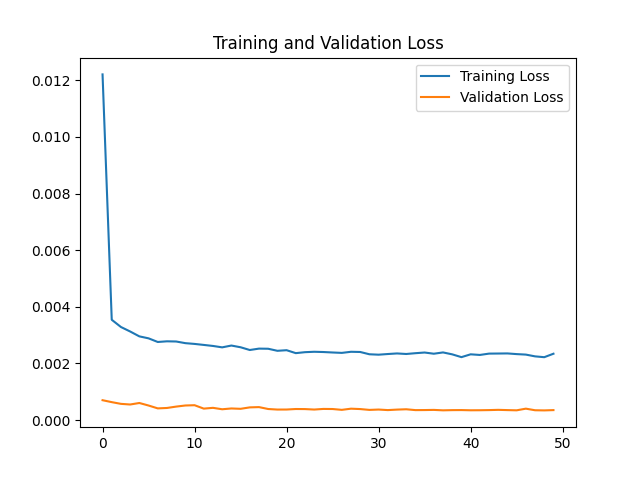测试集损失函数比训练集好很多的原因可能是因为加入了Dropout，训练时开启，丢掉一部分数据集，测试时Dropout关闭。
使用了10万行数据训练，1万测试，RMSE=0.03。
9.全部代码
import tensorflow as tf
from tensorflow_core import keras
import os
import pandas as pd
import numpy as np
import matplotlib.pyplot as plt
from sklearn.preprocessing import MinMaxScaler
from sklearn.metrics import mean_squared_error, r2_score
from numpy import concatenate

pd.set_option('display.max_columns', None) #显示所有列

'''修改数据'''
SaveFile_Path = r''  # 此处加入读取和保存的文件路径
savename = r''  #存储文件的名字
os.chdir(SaveFile_Path)  # 修改当前工作目录

DIR = 'LSTM'
if not os.path.exists(DIR):  #判断括号里的文件是否存在的意思，括号内的可以是文件路径。
os.makedirs(DIR)  #用于递归创建目录

TIME_STEP = 360 #时间步长360min 6h
DELAY = 10 #预测十分钟后
BATCHSZ = 5  #一个批处理(batch)大小
EACH_EPOCH = 3  #循环次数

'''
绘制初始数据曲线
'''
def plot_init():
values = df.values
col = df.shape
plt.rcParams['font.sans-serif'] = ['SimHei']  # 用来正常显示中文标签
plt.figure()
for i in range(0, col):
plt.subplot(col, 1, i+1)
plt.plot(values[:, i])
plt.title(df.columns[i], y=0.5, loc='right')
plt.show()

'''
LSTM数据准备
'''

def train_test(timestep, nextstep):   #timestep时间步长, nextstep预测多长时间
values = dataset.values
# ensure all data is float
values = values.astype('float32')
# normalize features归一化
scaler = MinMaxScaler(feature_range=(0, 1))  # sklearn 归一化函数
scaled = scaler.fit_transform(values)  # fit_transform(X_train) 意思是找出X_train的均值和​​​​​​​标准差，并应用在X_train上。
# split into train and test sets  将数据集进行划分，然后将训练集和测试集划分为输入和输出变量，最终将输入（X）改造为LSTM的输入格式，即[samples,timesteps,features]
n_train_hours = 2000 #20万行作训练集，11万作测试集
train = scaled[:n_train_hours, :]
test = scaled[n_train_hours:3000, :]

train_X = []
train_y = []
test_X = []
test_y = []

# 测试集：
# 利用for循环，遍历整个测试集，提取测试集中连续360min的特征量作为输入特征test_X，第361-370min的发电量作为标签
for i in range(len(train)-timestep-nextstep+1):
train_X.append(train[i:(i+timestep), :])
btemp = train[i+timestep:i+timestep+nextstep, 0]
b = []
for j in range(len(btemp)):
b.append(btemp[j])
train_y.append(b)

# 对训练集进行打乱
np.random.seed(7)
np.random.shuffle(train_X)
np.random.seed(7)
np.random.shuffle(train_y)
tf.random.set_seed(7)
# 将训练集由list格式变为array格式
train_X = np.array(train_X, dtype=np.float32)
train_y = np.array(train_y, dtype=np.float32)

# 使x_train符合RNN输入要求：[送入样本数， 循环核时间展开步数， 每个时间步输入特征个数]。
# 此处整个数据集送入，送入样本数为train_X.shape；输入360min数据，预测出第361min的发电量，循环核时间展开步数为360; 每个时间步送入的特征是某一min的运行数据，16个数据，故每个时间步输入特征个数为16
train_X = np.reshape(train_X, (train_X.shape, 360, 16))

# 测试集：
# 利用for循环，遍历整个测试集，提取测试集中连续360min的特征量作为输入特征test_X，第361-370min的发电量作为标签
for i in range(len(test)-timestep-nextstep+1):
test_X.append(test[i:(i + timestep), :])
btemp = test[i + timestep:i + timestep + nextstep, 0]
b = []
for j in range(len(btemp)):
b.append(btemp[j])
test_y.append(b)

test_X, test_y = np.array(test_X, dtype=np.float32), np.array(test_y, dtype=np.float32)
test_X = np.reshape(test_X, (test_X.shape, 360, 16))

#print(train_X.shape, train_y.shape, test_X.shape, test_y.shape)
return train_X, train_y, test_X, test_y, scaler

'''
构造模型
'''
def model_build(train_datas): #train_datas = train_X
# LSTM层
model = keras.models.Sequential()
model.add(keras.layers.LSTM(40, input_shape=(train_datas.shape[1:]), return_sequences=True, )) #, return_sequences=True 400记忆体个数
#配置训练方法

return model

'''模型拟合'''
def model_fit(model, train_datas, train_labels,x_test, y_test):    #train_X, train_y, test_X, test_y

checkpoint_save_path = "./checkpoint/LSTM_stock.ckpt" #模型保存位置

if os.path.exists(checkpoint_save_path + '.index'):

lr_reduce = keras.callbacks.ReduceLROnPlateau('val_loss',     #学习停止，模型会将学习率降低2-10倍，该hui
patience=4,
factor=0.7,
min_lr=0.00001)
best_model = keras.callbacks.ModelCheckpoint(filepath=checkpoint_save_path,#保存模型
monitor='val_loss',
verbose=0,
save_best_only=True,
save_weights_only=True,
mode='min',
)

early_stop = keras.callbacks.EarlyStopping(monitor='val_rmse', patience=15)

history = model.fit(
train_datas, train_labels,
validation_data=(x_test, y_test),
batch_size=BATCHSZ,
epochs=EACH_EPOCH,
verbose=2,
callbacks=[
best_model,
early_stop,
lr_reduce,
]
)

loss = history.history['loss']
val_loss = history.history['val_loss']
#画出训练集和测试集的损失函数
plt.plot(loss, label='Training Loss')
plt.plot(val_loss, label='Validation Loss')
plt.title('Training and Validation Loss')
plt.legend()
plt.savefig('./{}/{}.png'.format(DIR, '损失函数'))
plt.close()

return model, history

'''评价部分'''
def rmse(y_true, y_pred):  #sqrt求元素平方根  mean求张量平均值
return keras.backend.sqrt(keras.backend.mean(keras.backend.square(y_pred - y_true), axis=-1))

def model_evaluation(model, test_X, test_y, savename):
yhat = model.predict(test_X)
yhat = yhat[:, -1]    #只取最后一个时刻的进行分析，1-9min的结果不是很重要
y_normal = yhat.copy()
y_normal[y_normal < 0] = 0  #将预测值归一化中小于0的都置0
test_X = test_X[:, 0, :]
test_y = test_y[:, -1]   #对应取test_y最后一个时刻

#画出归一化后真实值和预测值的图像计算相关指标
model_plot(test_y, y_normal, '归一化')
RMSE_normal = np.sqrt(mean_squared_error(y_normal, test_y))
R2_SCORE_normal = r2_score(y_normal, test_y)
print('RMSE_normal: {}\nR2_SCORE_normal: {}\n'.format(RMSE_normal, R2_SCORE_normal))

#返回计算归一化前
yhat = yhat.reshape(len(yhat), 1)
inv_yhat = concatenate((yhat, test_X[:, 1:]), axis=1)
inv_y = scaler.inverse_transform(inv_yhat)  # 预测值转化
y_pred = inv_y[:, 0]   #预测值由归一化值转为真实值
y_pred[y_pred < 0] = 0  #将预测值真实值中小于0的都置0

test_y = test_y.reshape((len(test_y), 1))
inv_yact_hat = concatenate((test_y, test_X[:, 1:]), axis=1)
inv_y = scaler.inverse_transform(inv_yact_hat)
y_real = inv_y[:, 0]  # 标签由归一化值转为真实值
'''
在这里为什么进行比例反转，是因为我们将原始数据进行了预处理（连同输出值y），
此时的误差损失计算是在处理之后的数据上进行的，为了计算在原始比例上的误差需要将数据进行转化。
同时笔者有个小Tips：就是反转时的矩阵大小一定要和原来的大小（shape）完全相同，否则就会报错。
'''

#存储真实值和预测值的相关信息
y_pred_df = pd.DataFrame(index=y_pred)
y_pred_df.to_csv(r'./{}/LSTM_pred.csv'.format(DIR), encoding='gbk', sep=',')
y_real_df = pd.DataFrame(index=y_real)
y_real_df.to_csv(r'./{}/LSTM_real.csv'.format(DIR), encoding='gbk', sep=',')

# 画出真实值和预测值的图像并计算相关指标
model_plot(y_real, y_pred, savename)
RMSE = np.sqrt(mean_squared_error(y_pred, y_real))
R2_SCORE = r2_score(y_pred, y_real)
print('RMSE: {}\nR2_SCORE: {}\n'.format(RMSE, R2_SCORE))

return RMSE, R2_SCORE, RMSE_normal, R2_SCORE_normal

'''保存模型信息'''
def model_save(RMSE, R2_SCORE, RMSE_normal, R2_SCORE_normal, savename):
with open(r'./{}/LSTM.txt'.format(DIR), 'a') as fh:
fh.write('参数设置：\nTIME_STEP: {}\tDELAY: {}\n'.format(TIME_STEP, DELAY))
fh.write('RMSE: {}\nR2_SCORE: {}\n'.format(RMSE, R2_SCORE))
fh.write('RMSE_normal: {}\nR2_SCORE: {}\n\n'.format(RMSE_normal, R2_SCORE_normal))
print('%s模型信息保存成功！\n\n\n' % savename)

'''绘图相关'''
def model_plot(y_real, y_pred, savename):
plt.cla()
fig1 = plt.figure(figsize=(10, 14), dpi=80)

ax1 = fig1.add_subplot(1, 1, 1)  # 1行x1列的网格里第一个
ax1.plot(y_real, '-', c='blue', label='Real', linewidth=2)
ax1.plot(y_pred, '-', c='red', label='Predict ', linewidth=2)
ax1.legend(loc='upper right')
ax1.set_xlabel('min')
ax1.set_ylabel('KWH')
ax1.grid()

fig1.savefig('./{}/{}.png'.format(DIR, savename))
plt.close()

if __name__ == '__main__':
#plot_init()  画初始数据图像
train_X, train_y, test_X, test_y, scaler = train_test(timestep=360, nextstep=10)
'''模型拟合'''
model = model_build(train_X)
model, history = model_fit(model, train_X, train_y, test_X, test_y)
'''训练集评估'''
RMSE_list, R2_SCORE_list, RMSE_normal_list, R2_SCORE_normal_list = model_evaluation(model, train_X, train_y, '训练')

'''测试集评估'''
RMSE_list, R2_SCORE_list, RMSE_normal_list, R2_SCORE_normal_list = model_evaluation(model, test_X, test_y, '验证')
model_save(RMSE_list, R2_SCORE_list, RMSE_normal_list, R2_SCORE_normal_list, '验证')

10.总结
以上，来自刚入门的小菜鸡，如果有描述不当的地方，欢迎指正，问就是我错了。
其实写完之后再回看整个过程，发现代码本身其实并不难，但是对刚入门的新人来说，不会的东西太多了，调参都不知道该咋调，所以花了好多时间才写出来，希望这篇文章可以帮到同新入门的小白~
还有部分疑问：无法使用keras模型中的model.save(‘my_model.hdf5’)一直报错a bytes-like object is required, not ‘str’。
完整代码上传至了github：https://github.com/sw1122/LSTM.git
model.save无法保存.hdf5格式的问题已经解决，是tensorflow2.0配置文件的缺陷，tensorflow2.3,2.4版本已修复。缺少decode，解决方法如图：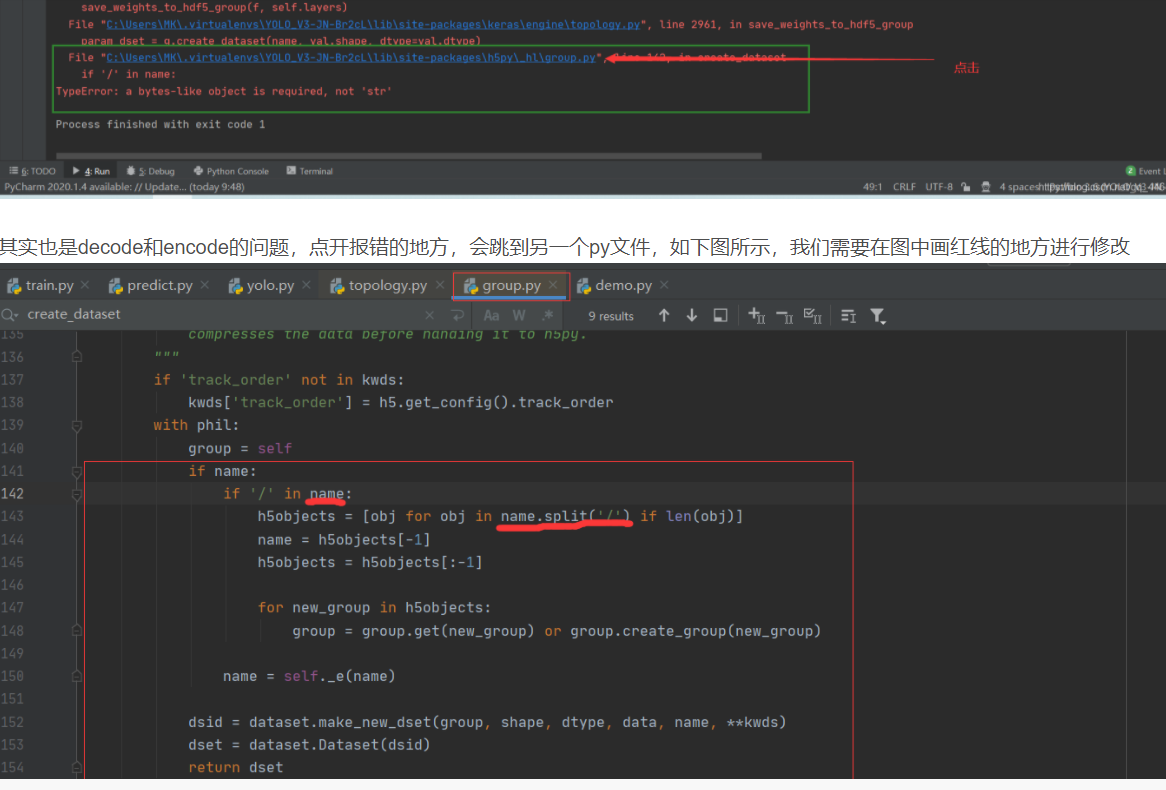详情可以参考这篇博客：https://blog.csdn.net/qq_44644355/article/details/109411624
展开全文python 深度学习 机器学习 tensorflow
• 相结合来预测轨迹。” 视觉计算国际研讨会。 斯普林格，湛，2019 年。 现在代码有点乱，我们正在做一些清理工作。 如果您有任何问题/查询，请发送电子邮件至。 此存储库包含 :check_mark: ETH 和 UCY 数据集的处理...
• 资源为今年八月份参加天池大数据竞赛a股公司营收预测使用的预处理后的数据和对应的算法文件
• 采用两年的历史数据作为训练集，2020年的数据作为测试集，最后采用2020年4月6日-4月19日的14天数据作为历史数据，去预测2020年4月20-27日的空气质量指数AQI。 输出结果已经打印出来，大家可以自行去查阅未来的...
摘要：
采用爬虫爬取了北京这个城市的空气质量指数日历史数据（爬取时间段为2018年1月1日至今天的前一天2020年4月19日），采用两年的历史数据作为训练集，2020年的数据作为测试集，最后采用2020年4月6日-4月19日的14天数据作为历史数据，去预测2020年4月20-27日的空气质量指数AQI。
输出结果已经打印出来，大家可以自行去查阅未来几天的AQI值进行比对。如果不准求不要骂我，不能怪我，我只是合理对模型进行了输入输出，不参与预测。
目录
摘要：
数据描述与处理：
模型构建与训练：
模型预测结果：
结论：
数据描述与处理：
AQI(Air Quality Index,空气质量指数)是报告每日空气质量的参数。
AQI是环境空气质量指数的缩写，是2012年3月国家发布的新空气质量评价标准，污染物监测为6项：二氧化硫、二氧化氮、PM10、PM2.5、一氧化碳和臭氧，数据每小时更新一次。AQI将这6项污染物用统一的评价标准呈现。
获取的数据为2018年1月1日至2020年4月19日，数据结构如图所示：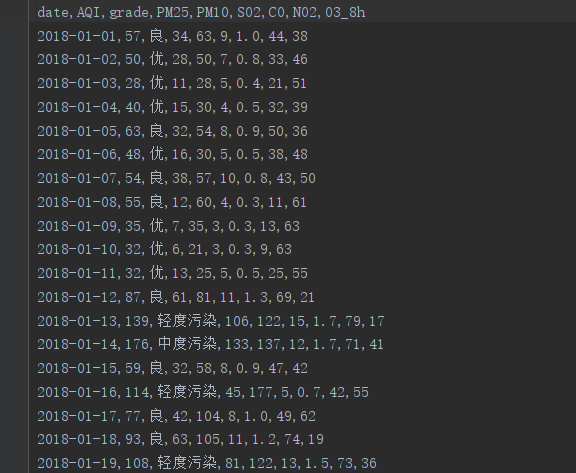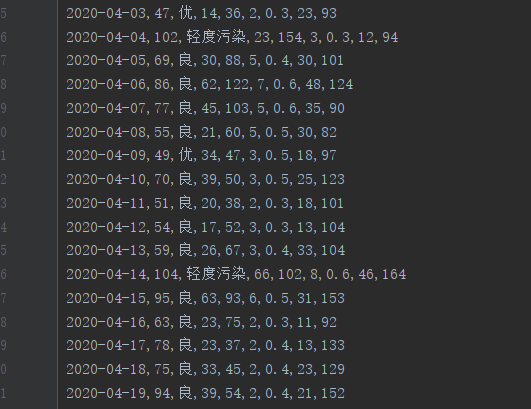模型构建与训练：
开始模型训练之前，无外乎要对LSTM模型构建时间序列的输入，本次尝试采用滑动窗口进行构建输入，核心代码如下：
def mult_data(dataset, target, start_index, end_index, history_size,target_size, step):
data = []
labels = []
start_index = start_index + history_size
if end_index is None:
end_index = len(dataset) - target_size
for i in range(start_index, end_index):
indices = range(i-history_size, i, step)
data.append(dataset[indices])
labels.append(target[i:i+target_size])
return np.array(data), np.array(labels)#返回输入数据特征和标签
LSTM模型核心代码如下：
multi_model = tf.keras.models.Sequential()
multi_model.compile(optimizer=tf.keras.optimizers.RMSprop(clipvalue=1.0), loss='mae')
通过迭代训练20次左右，观察损失函数图像如下图所示，大约在10次左右loss在测试集上已经降低最优了。于是改动了迭代次数及增加dropout防止过拟合，经过一系列调整之后，便可测试该模型的预测能力。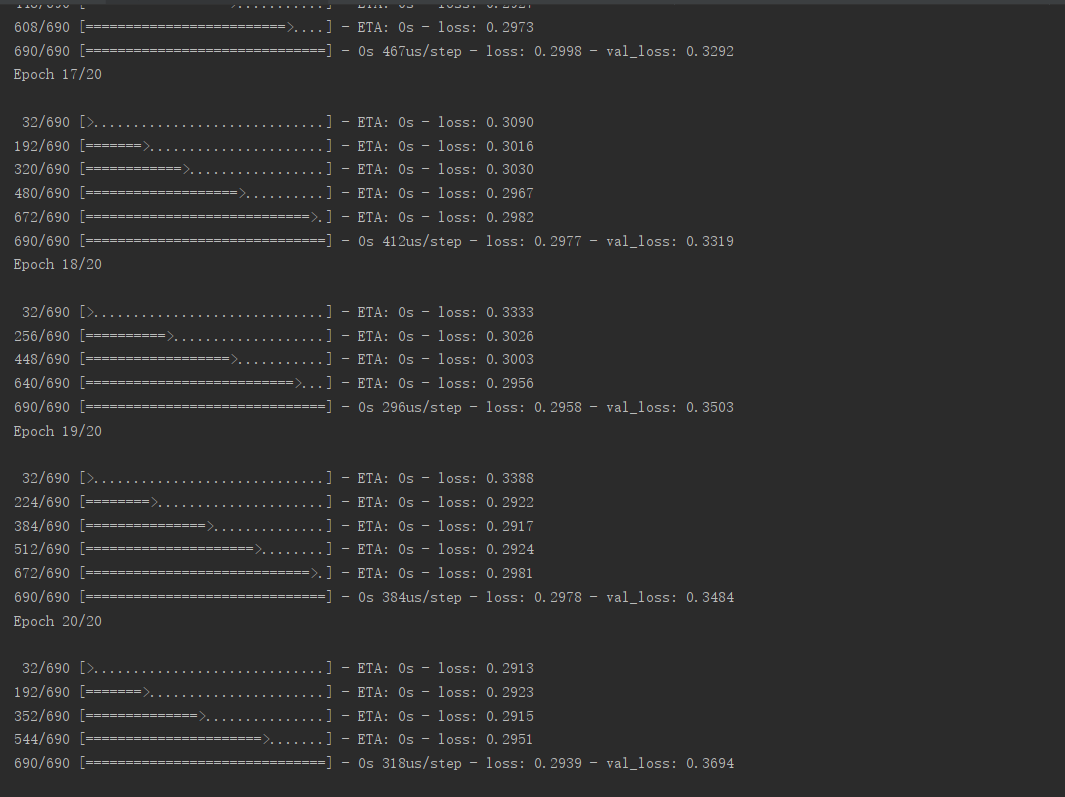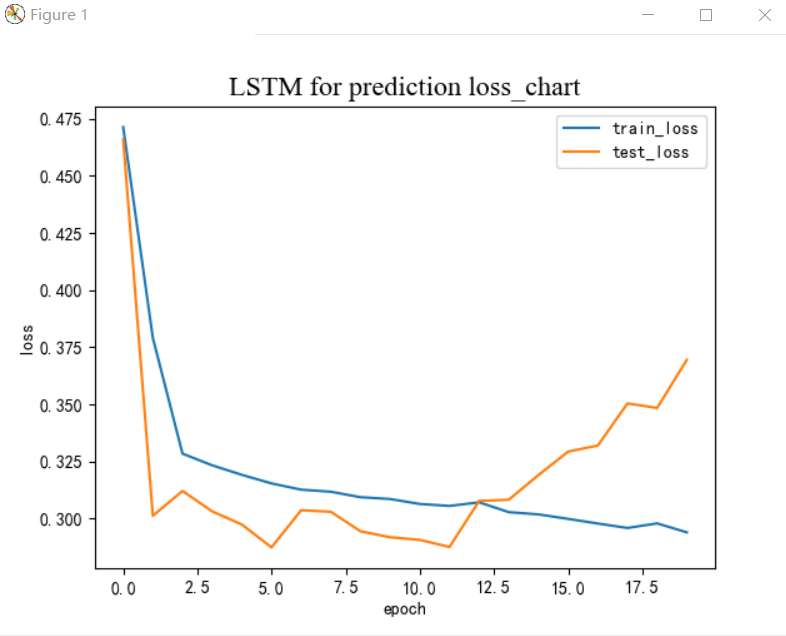模型预测结果：
按理说对预测结果值不能进行标准化（或者说归一化处理，因为均值和标准差只能使用训练数据来计算，但是为了能够显示输出，在这里不必那么纠结于细节）。对模型的输入为最近14天的数据（2020年4月6日-4月19日），输出为未来7天的预测结果。下图左边为输入数据展示，右边为模型输出展示，其中负号代表输入历史AQI数据值，输出的蓝色点为未来7天的AQI值；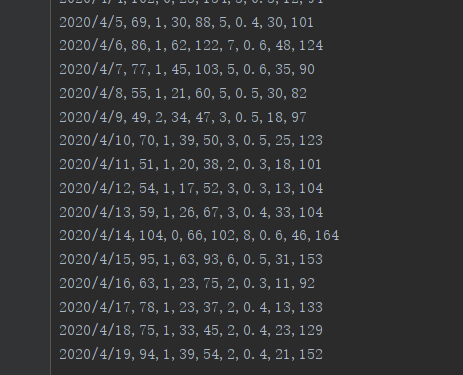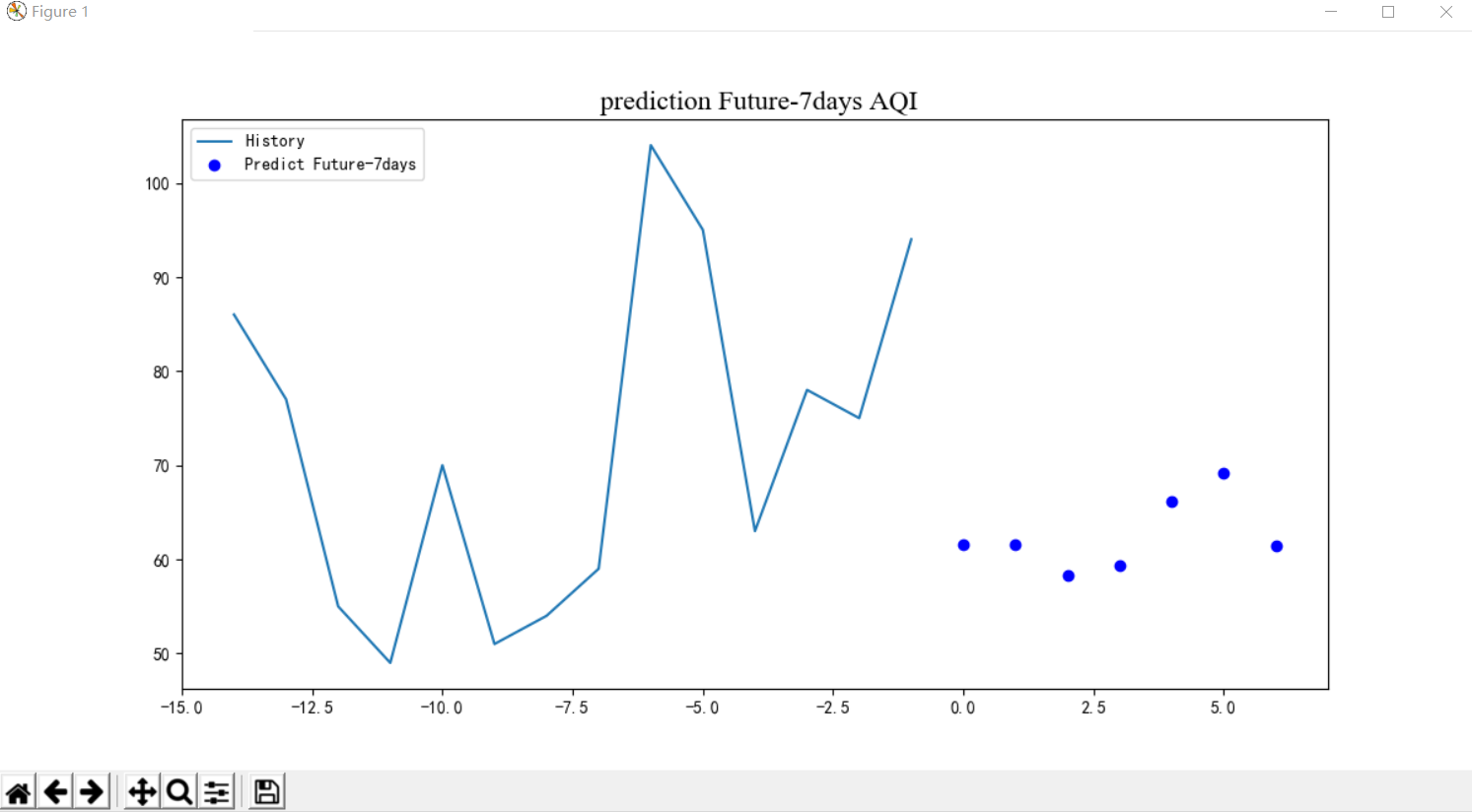为了方便大家看清，我在pycharm的输出面板打出了预测值，大家可以查阅。因为神经网络输出值不稳定，我找了几组比较好的结果展示出来，大家可以自行根据未来7天的北京AQI情况进行对比检验这个已经训练的LSTM模型的预测能力。查阅北京AQI数据的网站为：https://www.aqistudy.cn/historydata/daydata.php?city=北京
迭代10次的输出：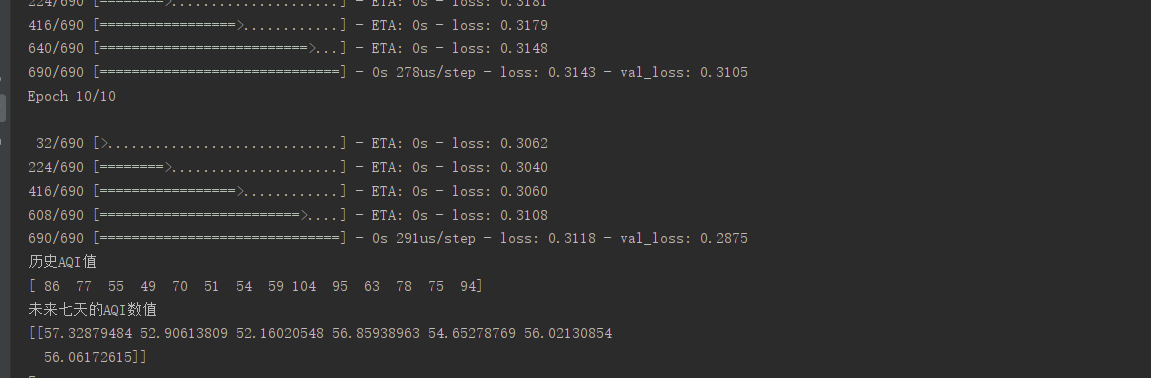迭代15次的输出：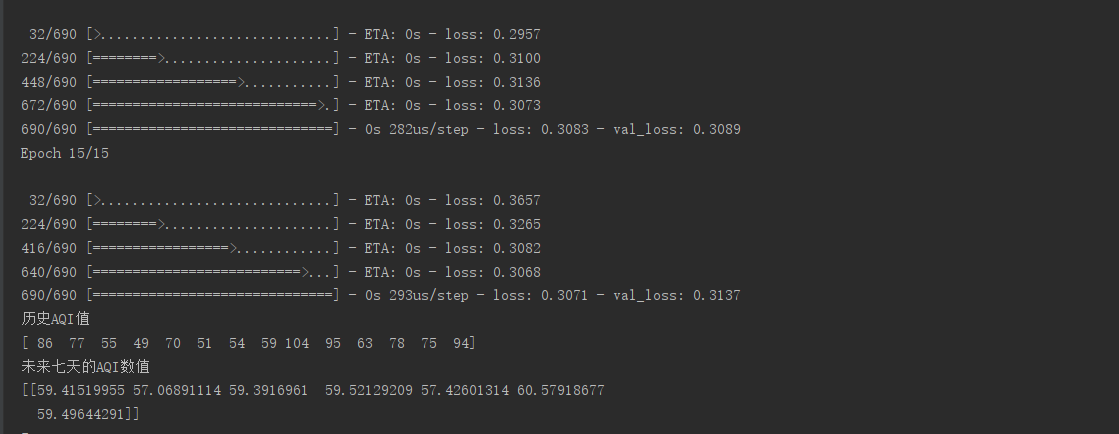结论：
通过预测出的结果发现，训练好的神经网络做线性预测还是很不稳定的，当然原因有很多，最明显的就是我对预测值结果进行了反归一化进行输出。但是，这个程序确实实现了未来7天AQI值的预测问题。在未来几天里，我将根据真实值与预测值之间的误差再次完善该方法。以求能够预测个大概趋势。如果大家有更好的解决思路，可以加Q525894654进行交流讨论。
接下来的几天便是等待验证结果的几天了。心情复杂且激动.....
展开全文人工智能 深度学习 神经网络
• 14通过对前14年的天气预报数据进行分析，（温度、风速，累计降雨量）预测未来6年的温度、风速和累计降雨量
• ## 利用LSTM预测时间序列未来........

万次阅读 多人点赞 2019-05-24 11:12:16
最近在做一个东西，用到了LSTM时间序列预测。。。 网上搜索吗，比如别人做过的比较典型的有： 1.https://blog.csdn.net/aliceyangxi1987/article/details/73420583 2....
ps.非常感谢各位大佬对这篇帖子的关注，我也没想到会这么多评论  - -！
已经写了很久啦，因为工作原因，后边可能不会来维护和回复评论了，谢谢。
这篇帖子只是提出了一点 lstm 的时间序列预测方法和疑问，抛砖引玉！

< ----------------- 正文-------------------------------- >

最近在做一个东西，用到了LSTM时间序列预测。。。
网上搜索吗，比如别人做过的比较典型的有：
1.  https://blog.csdn.net/aliceyangxi1987/article/details/73420583
2. https://www.cnblogs.com/mtcnn/p/9411597.html
3. https://cloud.tencent.com/developer/article/1083338  等等
他们都是利用已有的一条序列，当成Y ，再利用它往前滑动t-1构造一条序列X，
fit（X，Y）来做。以及后边利用测试集看表现啊 评估啊  等等。。。
然而，没有一个能够说利用LSTM如何真正预测未来的？？？
（因为按照他们这种做法，预测未来是没有这个所谓的 一个X  来喂给训练好的模型的？）

《《《-----------------------------滑动窗口的LSTM方法预测：----------------------------------------------------------------------------》》》
前面描述的都是比如一个时间序列的value为
Y ：[10, 20, 30, 40, 50, 60, 70, 80, 90]  他们会为其构造一条X  按照逐个t-1时刻的X对应逐个t时刻Y值。
X：[0, 10, 20, 30, 40, ......]      具体参考上述链接做法。
然后再利用构造的X，Y训练lstm模型，达到预测的目地。
但是这个做法，无法预测未来。

经过搜索，以上方法是数据LSTM中sequence to sequence 的方法的。一对一，适用于中英文翻译方法！！
或者一对一预测等等，不是预测未来的方法～ 所以前面那些链接中，应该是对sequence to sequence有点误会！
因此，我们应该采用滑动窗口的数据预测未来一个数据，sequence to vector的，可以根据前面几个预测下一个，比如下面：
比如X[t-3,t-2,t-1]   预测X[t],  此时X[t]可以当作标签Y
针对上述数据我们可以构造 (左为X， 右为Y )：
[10,20,30], 
[20,30,40], 
[30,40,50], 
.......................
这样依赖，利用多个滑动窗口（此处为3）来预测下一个数据的目地。。。。
预测未来如何逐个添加？ 答：唯有预测一个，往后添加一个，再加入预测。
缺点：误差会积累！

《《《-----------------------------滑动窗口的LSTM方法python实例：------------------------------------------------》》》
from numpy import array
from keras.models import Sequential
from keras.layers import LSTM
from keras.layers import Dense

'''
下面的split_sequence（）函数实现了这种行为，并将给定的单变量序列分成多个样本，其中每个样本具有指定的时间步长，输出是单个时间步。
'''
# split a univariate sequence into samples
def split_sequence(sequence, n_steps):
X, y = list(), list()
for i in range(len(sequence)):
# find the end of this pattern
end_ix = i + n_steps
# check if we are beyond the sequence
if end_ix > len(sequence)-1:
break
# gather input and output parts of the pattern
seq_x, seq_y = sequence[i:end_ix], sequence[end_ix]
X.append(seq_x)
y.append(seq_y)
return array(X), array(y)

if __name__ == '__main__':

# define input sequence
raw_seq = [10, 20, 30, 40, 50, 60, 70, 80, 90]
print raw_seq
# choose a number of time steps
n_steps = 3
# split into samples
X, y = split_sequence(raw_seq, n_steps)
print X, y
# reshape from [samples, timesteps] into [samples, timesteps, features]
n_features = 1
X = X.reshape((X.shape, X.shape, n_features))
# define model
model = Sequential()
model.add(LSTM(50, activation='relu', input_shape=(n_steps, n_features)))  # 隐藏层，输入，特征维
# fit model
model.fit(X, y, epochs=300, batch_size=1, verbose=2)  # 迭代次数，批次数，verbose决定是否显示每次迭代
# demonstrate prediction
x_input = array([70, 80, 90])
x_input = x_input.reshape((1, n_steps, n_features))
yhat = model.predict(x_input, verbose=0)
print x_input, yhat
print(yhat)
预测未来?
比如[70,80,90] 预测一个假如为
可以继续构造[80,90,100], 预测下一个。。。。
等等....
本文方法灵感参考转载于：
https://machinelearningmastery.com/how-to-develop-lstm-models-for-time-series-forecasting/
展开全文机器学习 深度学习
• lstm怎样实现对未来值的预测？ 所拥有的数据共3648个值，目标是继续向下预测3649，3650… 今天上午学习了https://blog.csdn.net/xspyzm ‘import_torch_as_tf’ 这位大佬写的。 总体思路就是：100个值预测一个，最后...
LSTM时间序列预测

文章目录
前言一、具体步骤1.导包、读取文件2.和上一篇的步骤一样3.新预测，向下预测7步4.画图
疑惑点

前言
lstm怎样实现对未来值的预测？ 所拥有的数据共3648个值，目标是继续向下预测3649，3650… 今天上午学习了https://blog.csdn.net/xspyzm ‘import_torch_as_tf’ 这位大佬写的。 总体思路就是：100个值预测一个，最后100个用做新的数据集再向下预测，预测一个添加到新数据集里，再向下预测一个。
一、具体步骤
1.导包、读取文件

import numpy as np
import matplotlib.pyplot as plt
import pandas as pd
import math
from keras.models import Sequential
from keras.layers import Dense
from keras.layers import LSTM
from sklearn.metrics import mean_squared_error
from sklearn.preprocessing import MinMaxScaler
from keras import backend as K
# 这个是报错Failed to convert a NumPy array to a Tensor ，然后搜了一下解决办法

dataframe = pd.read_csv(r'D:\PycharmProjects\ LSTM\hello3.csv',usecols = )
dataset = dataframe.values
plt.plot(dataset)
plt.show()


我的数据集：是不是有点多？？？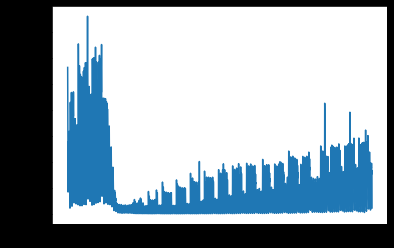2.和上一篇的步骤一样
def create_dataset(dataset,look_back = 100):
dataX,dataY = [],[]
for i in range(len(dataset)-look_back):    #  i 从 0 ：3548 ，dataX :0-100，1-101 ,dataY :100,101,102..
a = dataset[i:(i+look_back),0]
dataX.append(a)
dataY.append(dataset[i+look_back,0])
return np.array(dataX),np.array(dataY)

# 设置随机种子
np.random.seed(7)
scaler = MinMaxScaler(feature_range=(0,1))
# 数据集归一化
dataset = scaler.fit_transform(dataset)
train = dataset

# 设置时间滑窗，创建训练集

look_back = 100
trainX,trainY = create_dataset(train,look_back)    # train = dataset

# reshape trainX 成LSTM可以接受的输入 (样本，时间步，特征)
trainX = np.reshape(trainX,(trainX.shape,1,trainX.shape))

# 搭建lstm网络
model = Sequential()
# 输出节点为1，输入的每个样本的长度为look_back
model.fit(trainX,trainY,epochs = 100,batch_size = 1,verbose = 2)

# 预测训练集
trainPredict = model.predict(trainX)

不同点：没有划分训练集和测试集。直接将数据都作为了训练集，在后面预测时，算测试集。
3.新预测，向下预测7步
将原数据最后100个数据，添加到一个新数据里，然后预测一个，添加进去，直到预测7个。
testx = [0.]*(7+look_back)  # testx 为107个数据  列表
testx[0:look_back] = train[-look_back:]   #testx 0：100个数据是 train(dataset) 倒数100个
testx = K.cast_to_floatx(testx)
testx = np.array(testx)    # 把testx变为一个数组 ，二维(1,107)
testPredict = [0.]*7     # testPredict 是一个 7 个数据的列表
for i in range(7):
Xtest = testx[-look_back:]  # Xtest 是testx -100到最后的数据，共100个
Xtest = np.reshape(Xtest,(1,1,look_back))
testy = model.predict(Xtest)
testx[look_back+i] = testy
testPredict[i] = testy
testPredict = np.array(testPredict)
testPredict = np.reshape(testPredict,(7,1))

# 反标准化
trainPredict = scaler.inverse_transform(trainPredict)
trainY = scaler.inverse_transform([trainY])
testPredict = scaler.inverse_transform(testPredict)

# 输出RMSE
trainScore = math.sqrt(mean_squared_error(trainY[0,:],trainPredict[:,0]))
print('Train Score :%.2f RMSE'%(trainScore))

trainPredictPlot = np.reshape(np.array([None]*(len(dataset)+7)),((len(dataset)+7),1))
trainPredictPlot[look_back:len(trainPredict)+look_back,:] = trainPredict

testPredictPlot = np.reshape(np.array([None]*(len(dataset)+7)),((len(dataset)+7),1))
testPredictPlot[:, :] = np.nan
testPredictPlot[len(dataset):(len(dataset)+7), :] = testPredict

4.画图
plt.plot(scaler.inverse_transform(dataset) ,label='true')
plt.plot(trainPredictPlot,label='trainpredict')
plt.plot(testPredictPlot,label='testpredict')
plt.legend()  # 给图像加上图例
plt.show()

结果如下：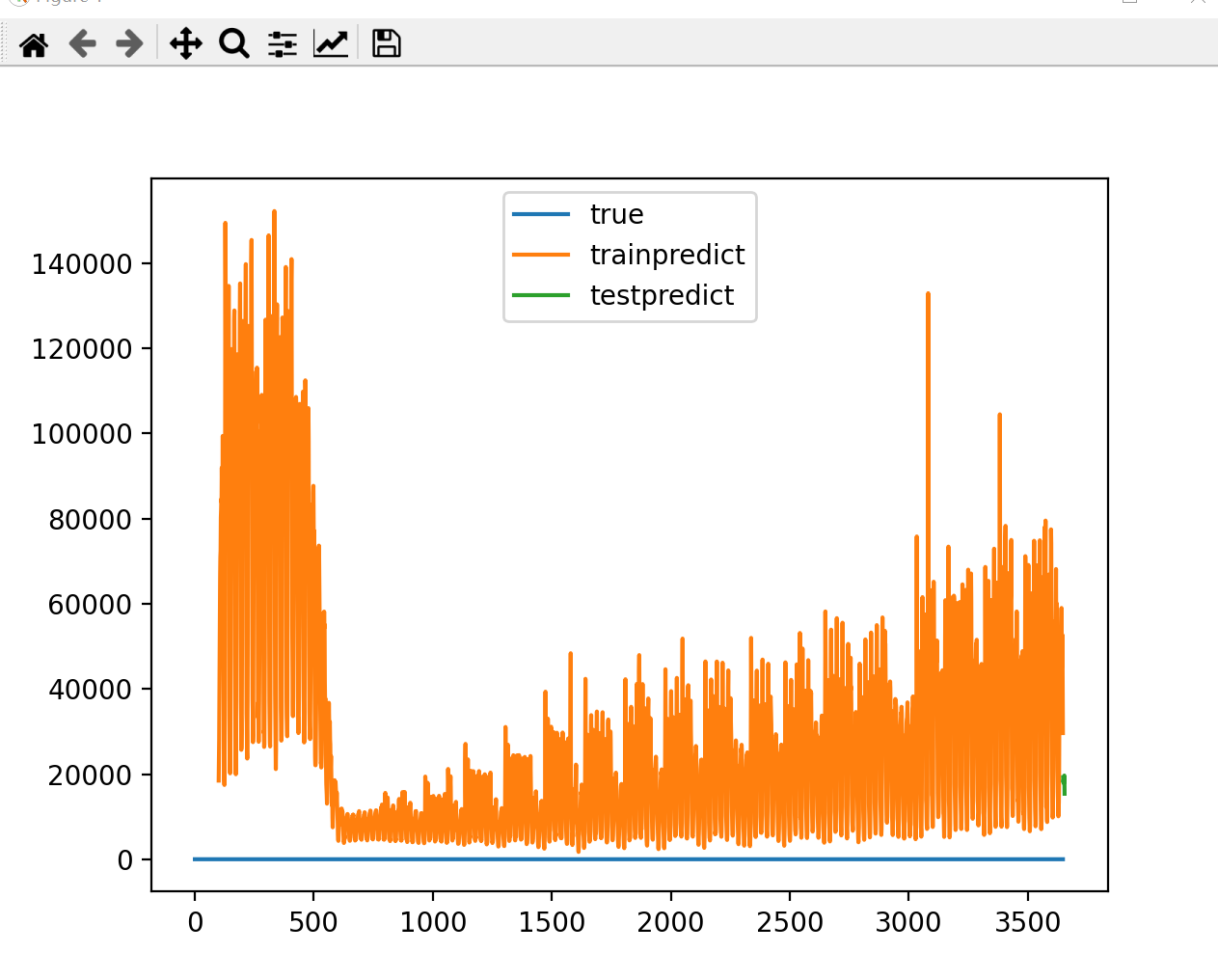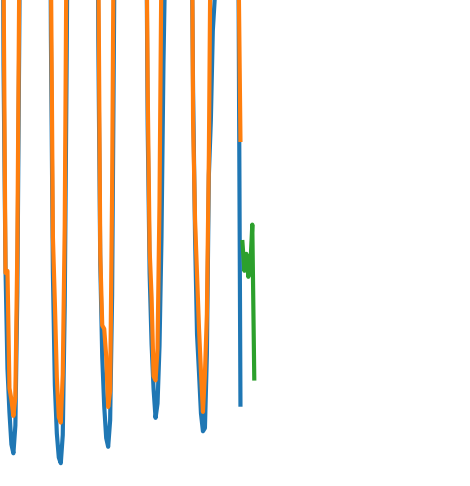疑惑点
感觉还是有点问题的。
LSTM是对前面时刻的相关信息选择保留还是遗忘，那这样子的话，就是预测的值只和前100个数据相关，其他数据无关。细节问题，在模型中各变量是怎么确定具体值得，怎样让训练结果更好。什么叫更好。 现在仅仅是跑出来了，需要学习的还有很多很多。
展开全文python 神经网络 机器学习
• 例如读取20的历史数据预测未来1的情况 batch_size = 535 # 训练批次大小，就是一次性读取多少个样本进行一运算，越大运算速度越快，但是占用内存和显存越大，根据自己的机器性能设置。同时该参数还决定梯度...
• lstm回归预测模型 加密货币和神经网络 (Cryptocurrency and Neural Network) Prediction of stock price is a quite challenging task due to volatile and non-linear nature of the financial stock markets. Here...机器学习 python 大数据 java 区块链
• 现在要预测未来7数据。有点不清楚具体该如何处理了。。求指点。。 个人想法：按照理论做法，需要事先反向提取最后7个数据，以预测第一个新值。然后利用第一个新值，并结合前面6个的数据，预测第二个新值，...python 人工智能 机器学习 深度学习 神经网络
• 注：本文章主要针对的是长周期的时间序列数据（10000-40000条为一个周期的数据预测 产生训练和测试数据 我们需要做的是产生周期为20000条/周期的sin函数时间序列（用于训练） 以及周期为40000条/周期的sin...时间序列
• 源程序代码 详解 股票预测 LSTM 时间序列rnn 代码程序数据集下载压缩包
• 在时间序列相关的很建模工作中，LSTM模型是经常会使用到的，从提出到现在LSTM模型已经有了很的扩展、变种和应用，今天我们简单地实现基于LSTM模型来对个变量的数据进行建模预测，在简单地预测中只能做单步预测...
• 文章目录数据格式代码部分 这个是我在github上看到的，学习完进行记录 数据格式 ... 使用LSTM算法对时间序列进行长期预测，标签是多维的，这里是二维，时间步长这里是12， 预测天数这里是10都可作时间序列
• 修改之后，新模型可以根据多天数据预测未来多天的结果。应用范围广泛，可以用于，股票预测，汇率预测，安全仓库预测，电力负荷预测等各种实际的应用。可以根据数据集的不同，使用该模型解决各种实际的预测问题。 ...python pycharm 开发语言
• 4个lstm数据预测的案例源代码，包括比特币行情预测、天气预报等
• LSTM模型的一个常见用途是对长时间序列数据进行学习预测，例如得到了某商品前一年的日销量数据，我们可以用LSTM模型来预测未来一段时间内该商品的销量。但对于不熟悉神经网络或者对没有了解过RNN模型的人来说，想要...
• 正如前面所说，如果你想用LSTM（或者说RNN）进行时间预测，那么比如必须将数据转化成LSTM可以接收的数据格式，这一点是不容置疑的；从LSTM自己的格式上也可以看出来；但是现在的疑惑在于，这种处理方式是正确的吗？...python 机器学习 人工智能 java 深度学习
• % if it is true the layer turn to the Bidirectional-LSTM and if it is false it will turn the units to the simple LSTM opt.isUseDropoutLayer = true; % dropout layer avoid of bieng overfit opt....matlab 机器学习
• 一、LSTM描述 长短期记忆网络（LSTM，Long Short-Term Memory）是一种时间循环神经网络，是为了解决一般的RNN（循环神经网络）存在的长期依赖问题而专门设计出来的，所有的RNN都具有一种重复神经网络模块的链式形式...MATLAB
• keras tensorflow lstm 变量序列的预测 + 数据文件，源代码
• 本文为你介绍如何在Keras深度学习库中搭建用于变量时间序列预测LSTM模型。 长短期记忆循环神经网络等几乎可以完美地模拟个输入变量的问题，这为时间序列预测带来极大益处。本文介绍了如何在 Keras 深度...
• 单变量时间序列预测 数据类型：单列 ​ import numpy import matplotlib.pyplot as plt from keras.models import Sequential from keras.layers import Dense from keras.layers import LSTM from keras....
• 本代码采用python语言写的一个LSTM时间序列来预测销量
• 最近，看到股票持续走低为我增强了信心，发现为行为...算法模型基于动态隐层LSTM RNN搭建，损失函数使用cross_entropy损失最大值，输入M维度、输出N维度，并预测长时间。代码基于Python3.6.X和Tensorflow1.13.X实现。神经网络 tensorflow 时序模型 行为分析
• 文章目录一、背景二、主要技术介绍1、RNN模型2、LSTM模型3、控制门工作原理四、代码实现五、案例分析六、参数设置七、结论 一、背景 近年来，股票预测还处于一个很热门的阶段，因为股票市场的波动十分巨大，随时...时间序列 python实现
• 本次比赛将使用LSTM模型来预测招商银行三后的收盘价，也就是利用5月10日前的数据，来预测5月15日的收盘价。 一、模型选择 股价价格的预测其实是一件极其不靠谱的事情。很专业机构和量化交易的个人都是极力在规避...
• Tensorflow中的LSTM自动编码器和LSTM未来预测器。 这是基于本文的简单实现： : 要求 Tensorflow 1.4.0 的Python 3.5.4 Python软件包：numpy，matplotlib，os，argparse，scipy 用法 数据生成后实施重建或未来预测......